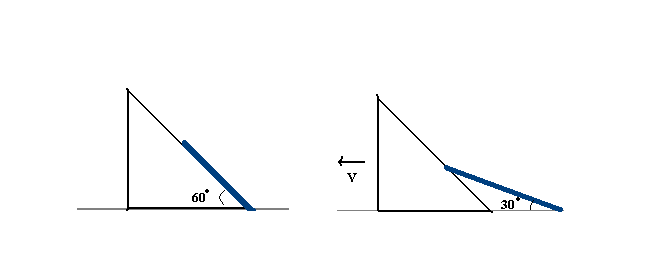# Which constraint would you use?A thin rod of mass $m = 1$ kg and length $l=1$ m is placed along the hypotenuse side of a wedge lying on a friction less floor having mass $M =5$ kg and angle $60°$. At $t= 0$ the system is released from rest. Both the objects are free to move. Find the speed of the wedge when the rod makes an angle $30°$ with the floor.

The answer can be expressed as $\sqrt { \frac { a(\sqrt { 3 } -1) }{ b } }$. Find $a+b$.

Notes

• Take $g=10m/{ s }^{ 2 }$
###### I got this question from Tanya Sharma.
×

Problem Loading...

Note Loading...

Set Loading...